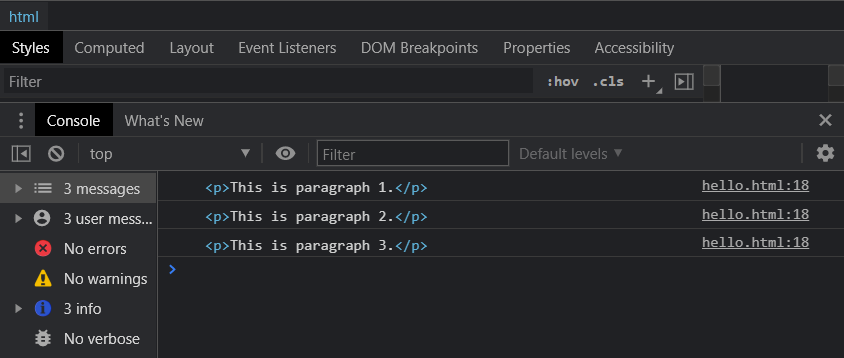Related Articles
How to loop through HTML elements without using forEach() loop in JavaScript ?
• Last Updated : 07 Apr, 2021

In this article, we will learn how to loop through HTML elements without using the forEach() method.

Approach 1: Using the for loop: The HTML elements can be iterated by using the regular JavaScript for loop. The number of elements to be iterated can be found using the length property. The for loop has three parts, initialization, condition expression, and increment/decrement expression. Each of the items can be accessed by using square brackets with their respective index.

Syntax:

```for (let i = 0; i < elements.length; i++) {
console.log(elements[i]);
}
```

Example:

## HTML

 ```<``html``>`` ` `<``body``>`` ` `    ````    ``<``p``>This is paragraph 1.``    ``<``p``>This is paragraph 2.``    ``<``p``>This is paragraph 3.`` ` `    ``<``script` `type``=``"text/javascript"``>``     ` `        ``// Get the elements to be iterated``        ``let htmlElements =``            ``document.getElementsByTagName("p");`` ` `        ``// Use a regular for-loop``        ``for (let i = 0; i < ``htmlElements.length``; i++) {`` ` `            ``// Print the current element``            ``console.log(htmlElements[i]);``        ``}``    `````` ` ``

Output:Approach 2: Using the While loop: The HTML elements can be iterated by using a regular JavaScript while loop. The number of elements can be found using the length property. A temporary value is used to keep track of the current iteration by checking it in the condition expression. Each of the items can then be accessed by using square brackets with their respective index.

Syntax:

```let i = 0;
while(i < elements.length) {
console.log(elements[i]);
i++;
}```

Example:

## HTML

 ```<``html``>`` ` `<``body``>`` ` `    ````    ``<``p``>This is paragraph 1.``    ``<``p``>This is paragraph 2.``    ``<``p``>This is paragraph 3.`` ` `    ``<``script` `type``=``"text/javascript"``>``     ` `        ``// Get the elements to be iterated``        ``let htmlElements =``            ``document.getElementsByTagName("p");`` ` `        ``// Define a variable to keep track``        ``// of the current iteration``        ``let i = 0;`` ` `        ``// Check if the current value``        ``// is lesser than total elements``        ``while (i < ``htmlElements.length``) {`` ` `            ``// Print the current element``            ``console.log(htmlElements[i]);`` ` `            ``// Increment the value``            ``i++;``        ``}``    `````` ` ``

Output:Approach 3: Using the ‘for…..of’ statement: The for…of statement can be used to loop over values of an iterable object. It includes objects like an Array, Map, Set, or HTML elements. A temporary variable holds the current value during the execution of the loop, which can then be used in the body of the loop.

Syntax:

```for (element of elements) {
console.log(element);
}```

Example:

## HTML

 ```<``html``>`` ` `<``body``>`` ` `    ````    ``<``p``>This is paragraph 1.``    ``<``p``>This is paragraph 2.``    ``<``p``>This is paragraph 3.`` ` `    ``<``script` `type``=``"text/javascript"``>``     ` `        ``// Get the elements to be iterated``        ``let HTMLelements =``            ``document.getElementsByTagName("p");`` ` `        ``// Use the for...of statement to get``        ``// the values``        ``for (elem of HTMLelements) {`` ` `            ``// Print the current element``            ``console.log(elem);``        ``}``    `````` ` ``

Output:My Personal Notes arrow_drop_up